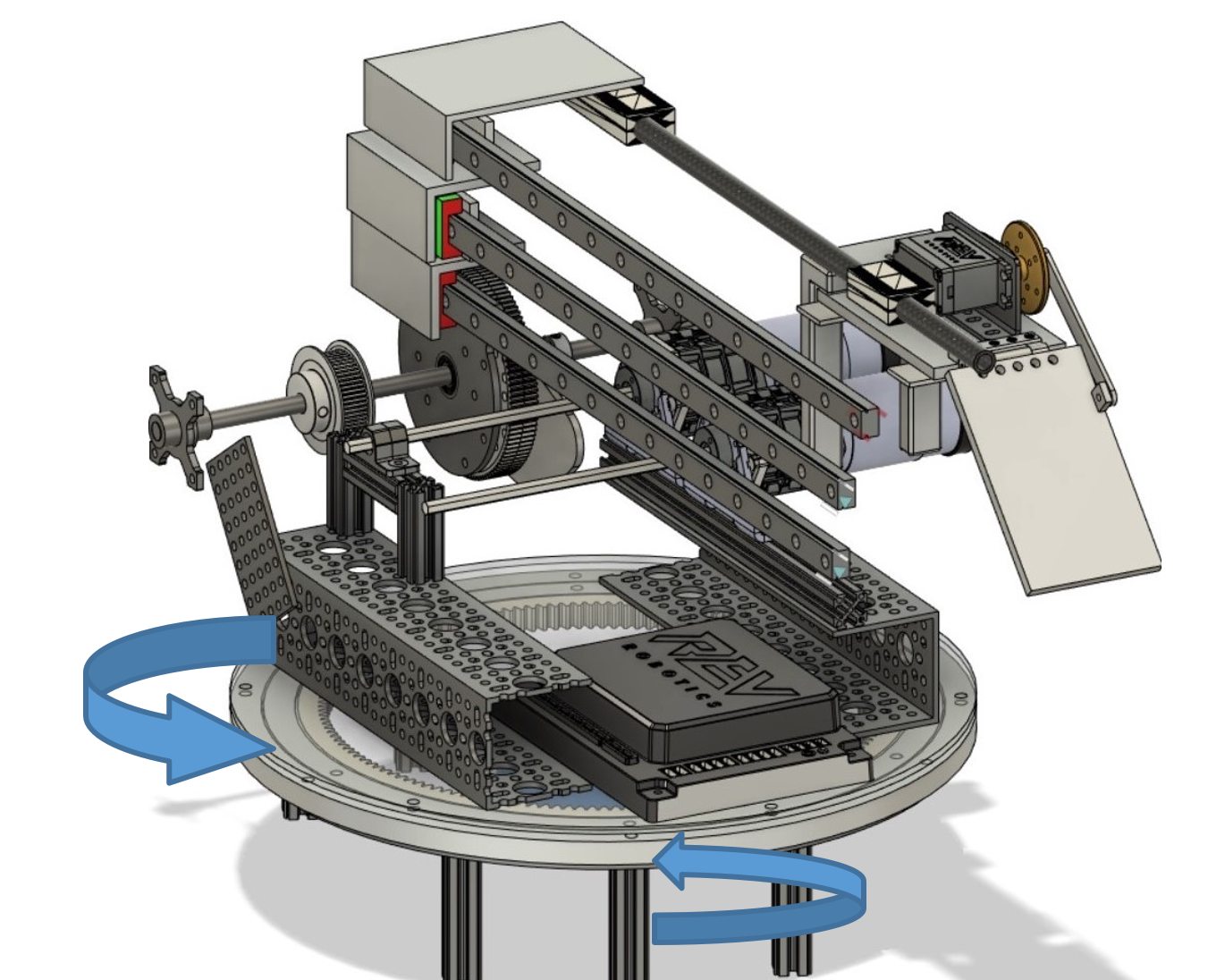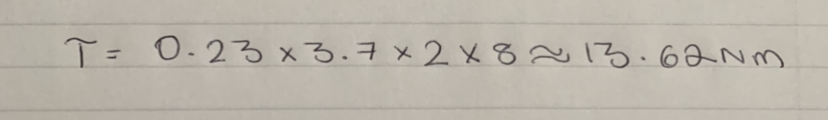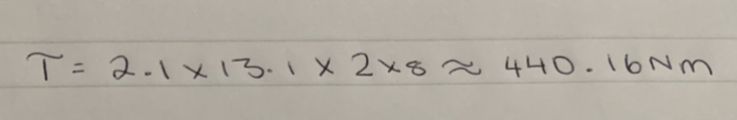# Calculating Torque at the Turn-table

Tags: think and design
Personhours: 5
Calculating Torque at the Turn-table By Bhanaviya

### Task: Analyze torque at the turn-table and how it affects our choice of motor setsWe want to know if we are using the best possible motor set on our turntable. Since our turn-table is programmed to rotate at the fastest possible speed, we are not too concerned with a motor that turns faster but rather one that has a higher level of control and produces a higher output torque. A faster turret gives us less control when we are turning on the field and we want to reduce the time we spend trying to get the turntable in the right orientation. So, it's time to replace its motors. Currently we are using a Neverest orbital motor on the bevel gear on the turret which has a stall torque of 0.23Nm. With a torque this low, we are not utilizing the turret to its maximum capability. We want to replace this with a REV HD Hex Motor with a double planetary cartridge. REV offers a manual which allows the user to see the exact gear ratios and output torque produced by each combination of cartridges. Based on the cartridges we have right now, two 4.1 cartridges have the highest output torque as per the manual. But we don't want to replace our current motor just yet, until we calculate the exact amount of torque and analyze whether this is a good amount.The gears operating the turntable are an 8:1 pinion gear and a 2:1 bevel gear. Thus, their compounded gear ratio is 16:1. Using this, we can find the output torque produced by our current motor which can be found by finding the value of 16 * 0.23Nm * 3.7 which gives us an output torque of 33.6. Now, this value can be higher so if we switch out with the double staged 4.1 HD Hex Motor, we get a compounded gear ratio of around 13.1, according to the REV manual. The stall torque for these motors are 2.1Nm which is significantly higher than the amount produced by the orbital motor. Plugging both these values and the 16:1 gear ratio of the turntable gears, we can find that the output torque produced by the turntable REV planetary motors can be found using the equation 16 * 2.1Nm * 13.1, which equates to 440.16 Nm.Based on these calculations, we can find that the planetary motors produce a significantly higher amount of torque and will give our turntable much more control and precision when operated. This amount of torque may cause some concern on how much the slower the turntable will be; as such, we also calculated the linear speed of the turret. To do this, we have to find the rotations per minute (RPM) of each motor. The no load RPM for the 3.7 motor is 1780 RPM. By dividing this by its gear ratio, we can find the RPM which is 1780 RPM/3.7Nm which is equal to 481.08m/s. As for the REV motor with more torque, its no-load RPM is 6000 RPM and when divided by its gear ratio of 13.1, we get a linear speed of 458.02m/s.Although this is a lower linear speed, the difference is very little, showing that the new 13.1 motors will not reduce our speed by much but will have a much larger effect on the output torque of the turret, thus maximizing the turntable's efficiency.

### Next Steps

Since we determined that our current turntable motor needed to be replaced, our next step was to make the physical switch on our bevel gear system on the turret. However, we are yet to test how much of an improvement the replacement will be so that will be our next goal. If we find that the motor set can be further replaced then we will use calculations similar to the ones in the article depending on whether we want to reduce or increase torque. Our next qualifier is this upcoming Saturday, so the new motors should improve our turntable control for the match-play portion.

Date | January 2, 2020